# Technology / Algorithms: Detecting Defects on Curved Edges

## Overview

For engineers who build manufacturing inspection systems for biomedical and consumer goods, the examples provided with this document are a flexible and powerful tool for detecting defects on curved edges. Unlike solutions using line scan cameras, the tools provided with this document are designed to be used with a broad variety of low-cost area scan cameras.

## Introduction

Detecting defects on straight edges is simple and straightforward with the analysis tools included in National Instruments Vision Software. The easiest way to perform this analysis is to use the “Find Straight Edge” tool and set a limit on the allowable deviation in pixels. For example, In Figure 1 below, the current deviation in pixels is 3.08. In order to classify the deviation in Figure 1 as a defect, the user should set the allowable deviation to 3.00 (pix).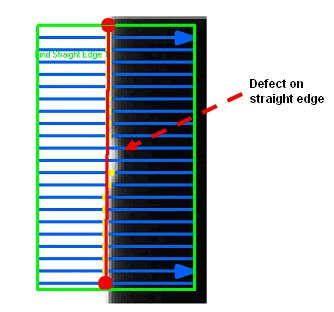Figure 1: Find Straight Edge with defect detection

However, when inspecting for defects on a curved edge, calculating the deviation from a straight edge is not sufficient.

Curved edges are present in many applications. A typical example is using a vision system to inspect an object that is moving across a roller (newspaper, textile fabric, latex rubber, etc.). Figure 2 below shows a typical example of a such application. This is a textile fabric that is being inspected for defects on the left and right edges. The image is taken with a standard areascan camera, and due to the parallax phenomenon, objects further away from the camera lens appear smaller than objects closer to the lens. This problem could in this case be solved by utilizing an expensive telecentric lens, but it would require two cameras (and hence two telecentric lenses) since the engineer would need to test the two edges individually: Telecentric lenses need to be as large as the device under test.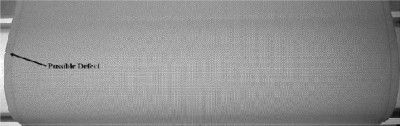Figure 2: Find Straight Edge with defect detection

Another solution to the problem above would be to use a linescan camera. This is also the only option available if the object is moving very fast (See Figure 3 below):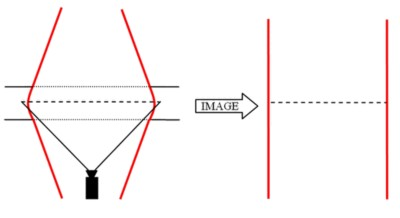Figure 3: Inspecting an object that is moving across the camera on a roller is typically performed with a linescan camera.

If the object is moving slowly or if inspection time permits the movement of the material to be stopped, using an area scan camera is much less expensive.

This paper will describe two methods for detecting these defects on curved edges: Polynomial fits and slope determination.

## Techniques

The best straight line fit utilizes a first order tool. For circular edges, the standard deviation from a second order circular fit can be used as an indicator of the severity of the edge defects. When the edges are non-linear or non-circular, one method for detecting defects along the edge is to apply a higher order polynomial model of the edge. For example, a curved edge can be modeled with a 3rd or higher order polynomial fit. A defect is indicated if the maximum difference between the polynomial curve and the original data is larger than a set number of standard deviations. In LabVIEW, this calculation can be performed using the rake tool to measure the position of the edge points. Then, we use the edge points to detect deviations from the best fit n-th order polynomial fit (Figure 4).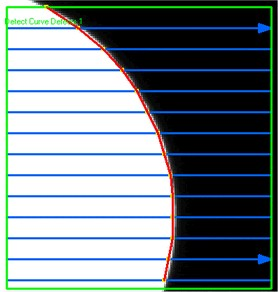Figure 4: Using rake tool to get edge points along curved edge.

A second technique that can be used to detect defects on curved edges is to measure the slope between adjacent edge points. Using this method, a defect is indicated if the slope exceeds a specified limit.

Both the polynomial fit and the slope method requires a rake function to collect the coordinates of points along curved edge. To ensure sufficient precision in detecting defects on the edge, the gap between each individual line on the rake function must be set to no more than half the size of the detectable defect size.

## Implementation

Standard Deviation Method

Implementation of the standard deviation method for curved edge detection is performed with the following pseudo code:

START
Find Edge Coordinates with Rake Tool
Calculate best polynomial fit through Edge Coordinates
For eace Line used with the Rake Tool, calculate distances between best polynomial fit intersection and original edge found on line
If Largest Distance larger than acceptable number of Standard Deviations
Edge Quality = FALSE (Defect Found)
If Largest Distance smaller than or equal to acceptable number of Standard Deviations
Edge Quality = True (Defect not Found)
END

The following figure shows the LabVIEW Diagram implementation of the polynomial fit / standard deviation method.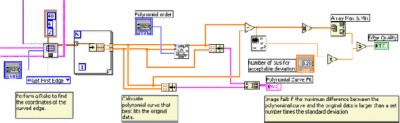Figure 5: LabVIEW diagram for detecting defects on a curved edge using the standard deviation from the Polynomial Fit.

As mentioned above, the rake tool is utilized to measure the coordinates of points along the curved edge. Then, the polynomial fit VI creates the best curve fit to these points. To detect defects, the location of each point of the polynomial curve is compared with the points measured by the rake tool. If a curve and edge points on the same rake line is further apart than a specified number of standard deviations, the inspection fails.

The following two figures illustrate this implementation inspecting a curved edge of a textile fabric.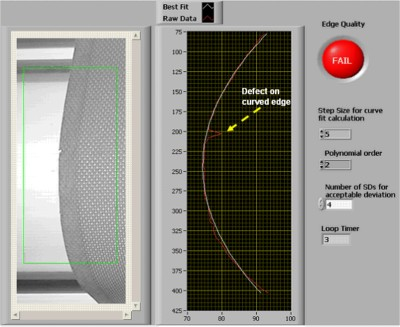Figure 6: Detecting defects on a curved edge using standard deviation from the Polynomial Fit.

In figure 6, the inspection fails because at the location of the defect the difference between the polynomial curve and the original data is above 4 standard deviations.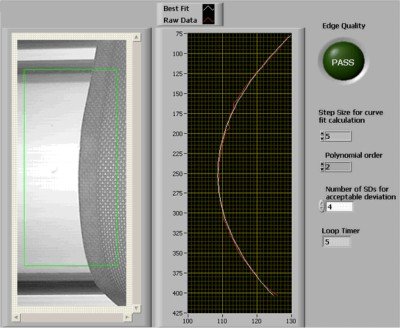Figure 7: Detecting defects on a curved edge using standard deviation from the Polynomial Fit.

In Figure 7, the inspection passes because all the measured edge points fall within 4 standard deviations of the polynomial curve.

Slope Method

Implementation of the slope method for curved edge detection is performed with the following pseudo code:

START
Find Edge Coordinates with Rake Tool
For each neighboring pair of Edge Coordinates Points
Calculate Slope of line connecting Edge Coordinate Points (point i=0 to n)
If i >0 and Slope > "Max Slope", Pass/Fail = FALSE (Defect Found)
EXIT
If i >0 and Slope <= "Max Slope", Pass/Fail = True (Defect Not Found)
If i = number of edges found
EXIT
END

The following figure shows the LabVIEW Diagram implementation of the slope method.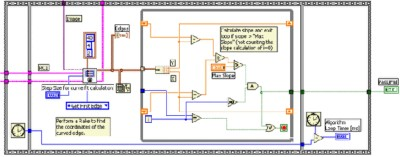Figure 8: LabVIEW diagram for detecting defects on a curved edge using slope deviations.

As with the polynomial fit method, the rake tool is utilized to collect the coordinates of points along the curved edge. Then the slope between adjacent points is calculated and compared to a specified maximum value.

Figure 9 shows this VI detecting defects on a curved edge of a textile fabric.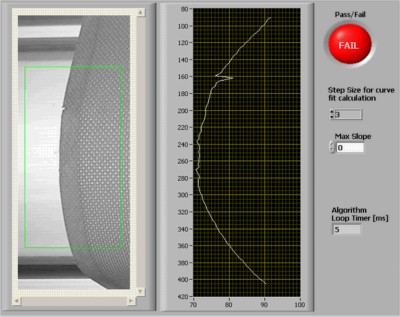Figure 9: Detecting defects on a curved edge using slope deviations.

In Figure 9 the inspection fails because the slope is more than the Max Slope

## Performance

Both the polynomial- and the slope-based curved edge defect detection algorithms run consistently faster than 10ms. The average time for the polynomial based method is about 3 ms and the slope detection method is about 5 ms on a 1GHz computer system.

## Conclusion

It has been shown that by using relatively simple NI LabVIEW Vision software tools, engineers and scientists can perform detections of defects on any curved edge.